# Biconditional Statements 2 4 and Definitions Warm Up

• Slides: 29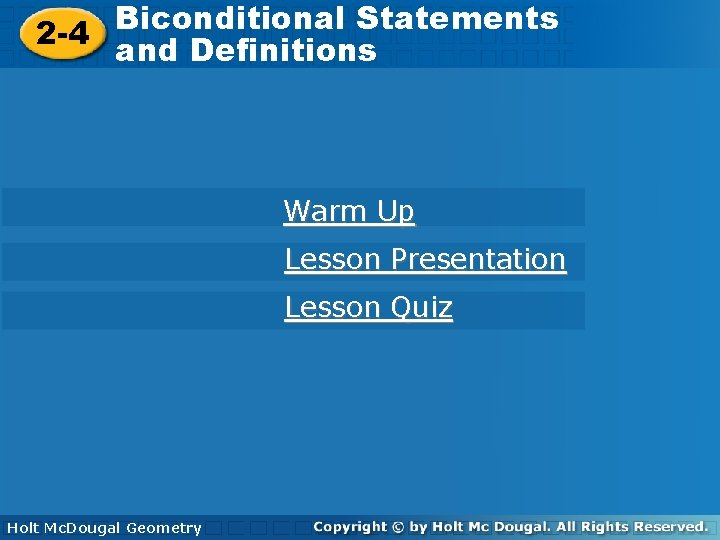Biconditional Statements 2 -4 and Definitions Warm Up Lesson Presentation Lesson Quiz Holt Geometry Holt Mc. Dougal GeometryBiconditional Statements 2 -4 and Definitions Warm Up Write a conditional statement from each of the following. 1. The intersection of two lines is a point. If two lines intersect, then they intersect in a point. 2. An odd number is one more than a multiple of 2. If a number is odd, then it is one more than a multiple of 2. 3. Write the converse of the conditional “If Pedro lives in Chicago, then he lives in Illinois. ” Find its truth value. If Pedro lives in Illinois, then he lives in Chicago; False. Holt Mc. Dougal Geometry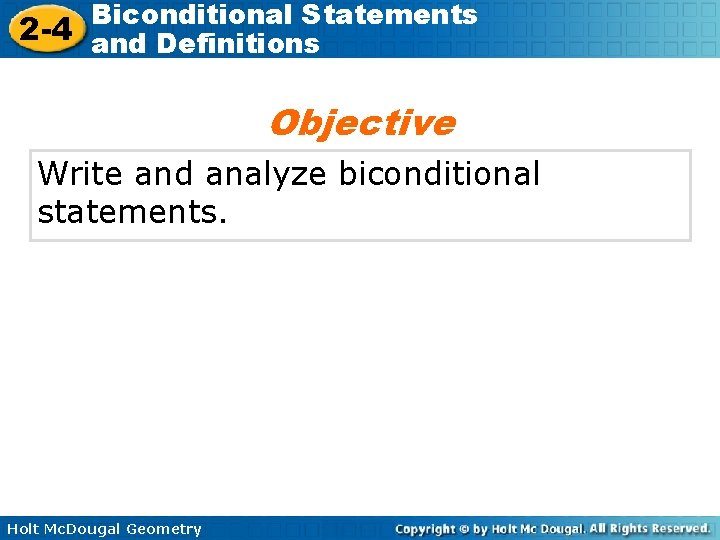Biconditional Statements 2 -4 and Definitions Objective Write and analyze biconditional statements. Holt Mc. Dougal GeometryBiconditional Statements 2 -4 and Definitions Vocabulary biconditional statement definition polygon triangle quadrilateral Holt Mc. Dougal Geometry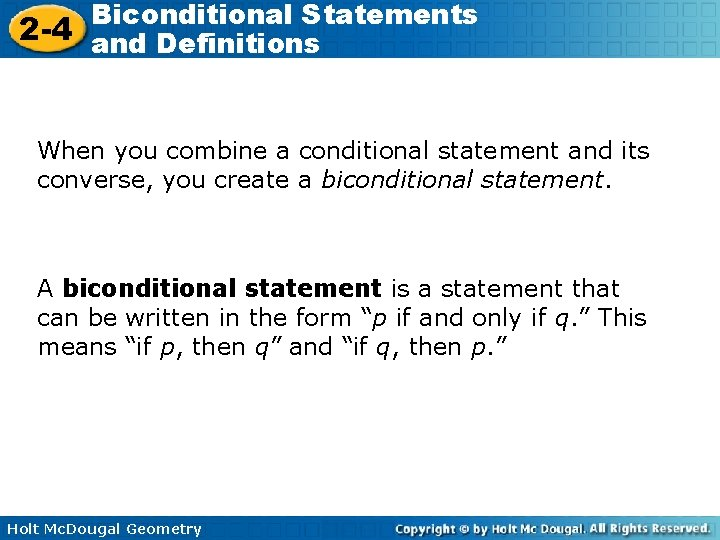Biconditional Statements 2 -4 and Definitions When you combine a conditional statement and its converse, you create a biconditional statement. A biconditional statement is a statement that can be written in the form “p if and only if q. ” This means “if p, then q” and “if q, then p. ” Holt Mc. Dougal GeometryBiconditional Statements 2 -4 and Definitions p q means p q and q p Writing Math The biconditional “p if and only if q” can also be written as “p iff q” or p q. Holt Mc. Dougal GeometryBiconditional Statements 2 -4 and Definitions Example 1 A: Identifying the Conditionals within a Biconditional Statement Write the conditional statement and converse within the biconditional. An angle is obtuse if and only if its measure is greater than 90° and less than 180°. Let p and q represent the following. p: An angle is obtuse. q: An angle’s measure is greater than 90° and less than 180°. Holt Mc. Dougal GeometryBiconditional Statements 2 -4 and Definitions Example 1 A Continued Let p and q represent the following. p: An angle is obtuse. q: An angle’s measure is greater than 90° and less than 180°. The two parts of the biconditional p q are p q and q p. Conditional: If an is obtuse, then its measure is greater than 90° and less than 180°. Converse: If an angle's measure is greater than 90° and less than 180°, then it is obtuse. Holt Mc. Dougal Geometry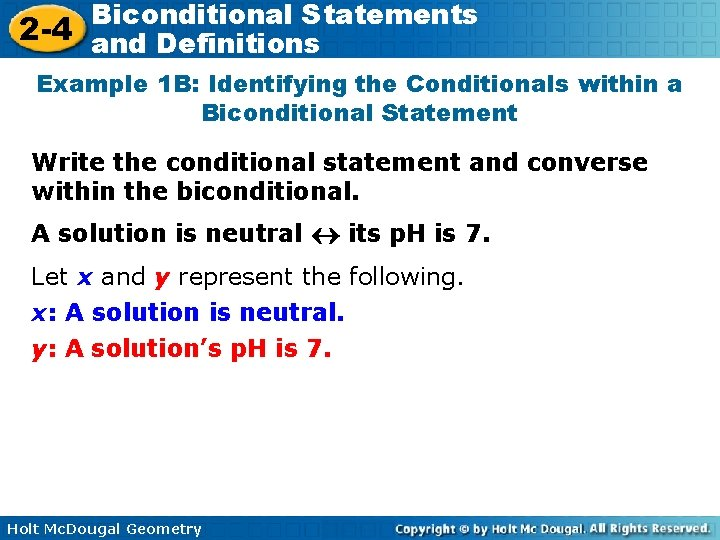Biconditional Statements 2 -4 and Definitions Example 1 B: Identifying the Conditionals within a Biconditional Statement Write the conditional statement and converse within the biconditional. A solution is neutral its p. H is 7. Let x and y represent the following. x: A solution is neutral. y: A solution’s p. H is 7. Holt Mc. Dougal GeometryBiconditional Statements 2 -4 and Definitions Example 1 B Continued Let x and y represent the following. x: A solution is neutral. y: A solution’s p. H is 7. The two parts of the biconditional x y are x y and y x. Conditional: If a solution is neutral, then its p. H is 7. Converse: If a solution’s p. H is 7, then it is neutral. Holt Mc. Dougal Geometry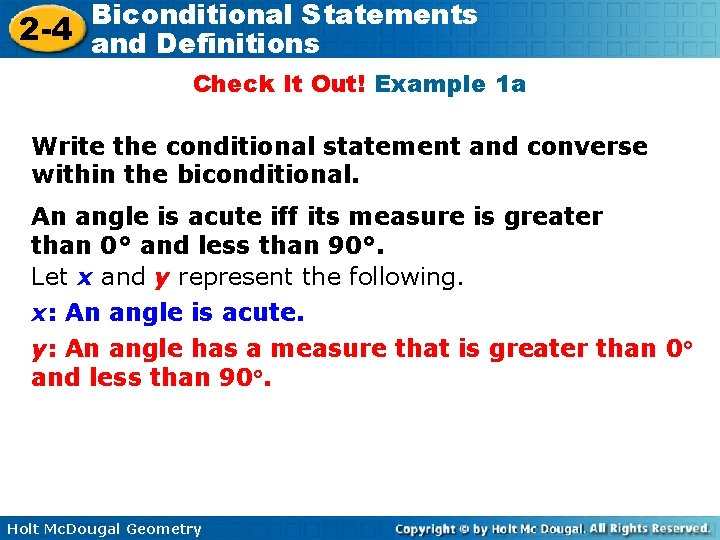Biconditional Statements 2 -4 and Definitions Check It Out! Example 1 a Write the conditional statement and converse within the biconditional. An angle is acute iff its measure is greater than 0° and less than 90°. Let x and y represent the following. x: An angle is acute. y: An angle has a measure that is greater than 0 and less than 90. Holt Mc. Dougal Geometry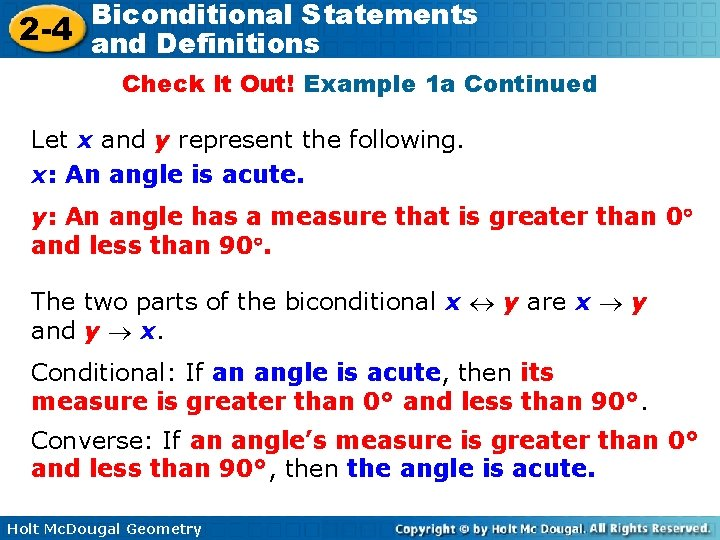Biconditional Statements 2 -4 and Definitions Check It Out! Example 1 a Continued Let x and y represent the following. x: An angle is acute. y: An angle has a measure that is greater than 0 and less than 90. The two parts of the biconditional x y are x y and y x. Conditional: If an angle is acute, then its measure is greater than 0° and less than 90°. Converse: If an angle’s measure is greater than 0° and less than 90°, then the angle is acute. Holt Mc. Dougal GeometryBiconditional Statements 2 -4 and Definitions Check It Out! Example 1 b Write the conditional statement and converse within the biconditional. Cho is a member if and only if he has paid the \$5 dues. Let x and y represent the following. x: Cho is a member. y: Cho has paid his \$5 dues. The two parts of the biconditional x y are x y and y x. Conditional: If Cho is a member, then he has paid the \$5 dues. Converse: If Cho has paid the \$5 dues, then he is a member. Holt Mc. Dougal Geometry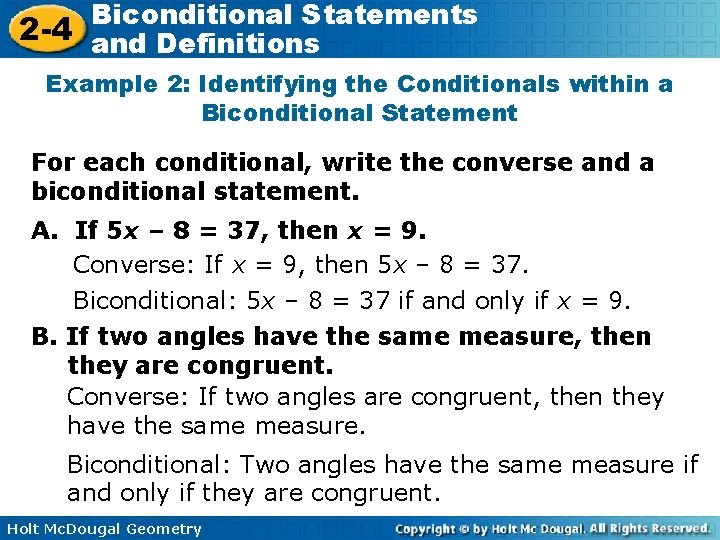Biconditional Statements 2 -4 and Definitions Example 2: Identifying the Conditionals within a Biconditional Statement For each conditional, write the converse and a biconditional statement. A. If 5 x – 8 = 37, then x = 9. Converse: If x = 9, then 5 x – 8 = 37. Biconditional: 5 x – 8 = 37 if and only if x = 9. B. If two angles have the same measure, then they are congruent. Converse: If two angles are congruent, then they have the same measure. Biconditional: Two angles have the same measure if and only if they are congruent. Holt Mc. Dougal Geometry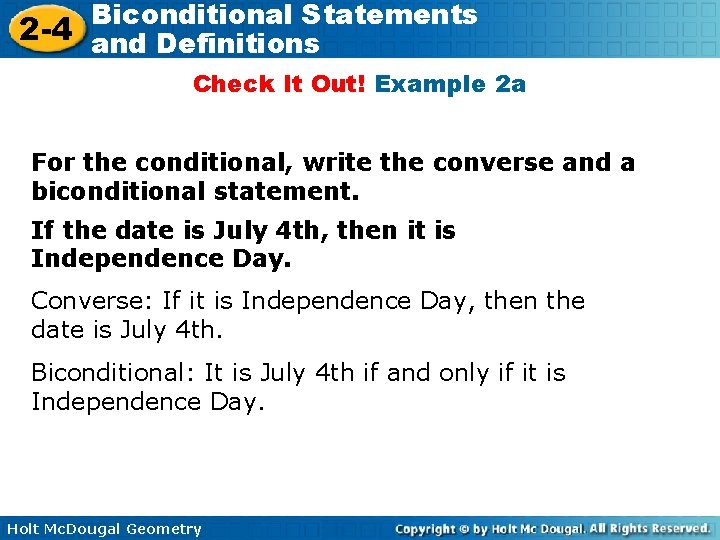Biconditional Statements 2 -4 and Definitions Check It Out! Example 2 a For the conditional, write the converse and a biconditional statement. If the date is July 4 th, then it is Independence Day. Converse: If it is Independence Day, then the date is July 4 th. Biconditional: It is July 4 th if and only if it is Independence Day. Holt Mc. Dougal GeometryBiconditional Statements 2 -4 and Definitions Check It Out! Example 2 b For the conditional, write the converse and a biconditional statement. If points lie on the same line, then they are collinear. Converse: If points are collinear, then they lie on the same line. Biconditional: Points lie on the same line if and only if they are collinear. Holt Mc. Dougal Geometry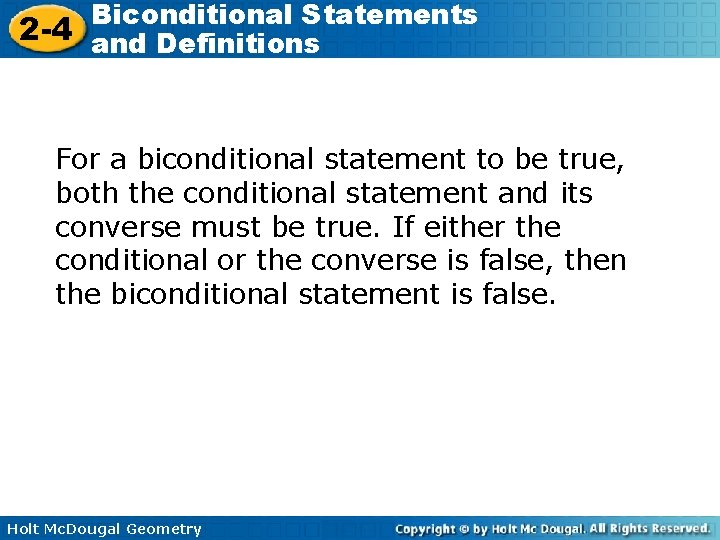Biconditional Statements 2 -4 and Definitions For a biconditional statement to be true, both the conditional statement and its converse must be true. If either the conditional or the converse is false, then the biconditional statement is false. Holt Mc. Dougal GeometryBiconditional Statements 2 -4 and Definitions Example 3 A: Analyzing the Truth Value of a Biconditional Statement Determine if the biconditional is true. If false, give a counterexample. A rectangle has side lengths of 12 cm and 25 cm if and only if its area is 300 cm 2. Holt Mc. Dougal Geometry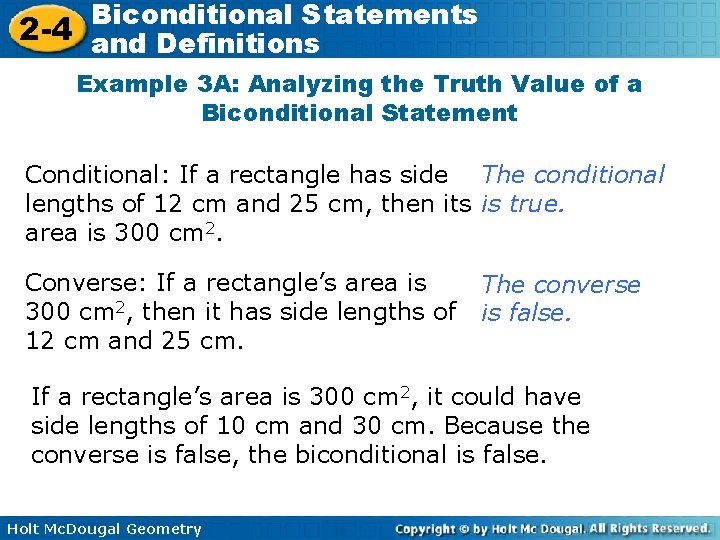Biconditional Statements 2 -4 and Definitions Example 3 A: Analyzing the Truth Value of a Biconditional Statement Conditional: If a rectangle has side The conditional lengths of 12 cm and 25 cm, then its is true. area is 300 cm 2. Converse: If a rectangle’s area is 300 cm 2, then it has side lengths of 12 cm and 25 cm. The converse is false. If a rectangle’s area is 300 cm 2, it could have side lengths of 10 cm and 30 cm. Because the converse is false, the biconditional is false. Holt Mc. Dougal Geometry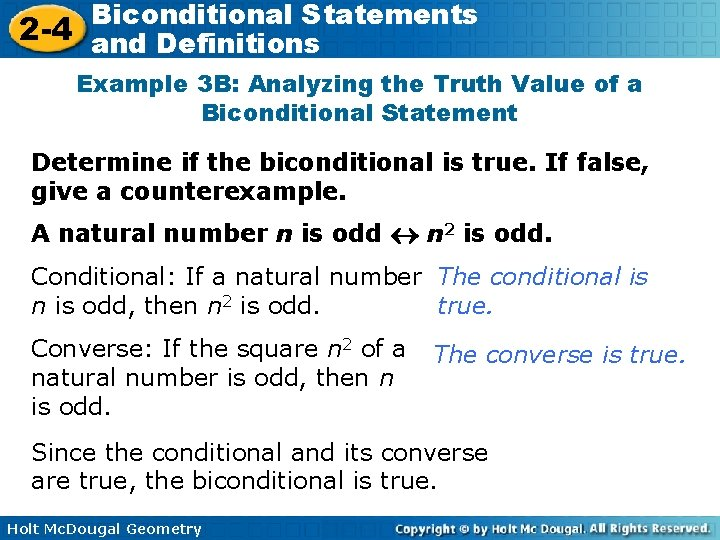Biconditional Statements 2 -4 and Definitions Example 3 B: Analyzing the Truth Value of a Biconditional Statement Determine if the biconditional is true. If false, give a counterexample. A natural number n is odd n 2 is odd. Conditional: If a natural number The conditional is n is odd, then n 2 is odd. true. Converse: If the square n 2 of a natural number is odd, then n is odd. The converse is true. Since the conditional and its converse are true, the biconditional is true. Holt Mc. Dougal GeometryBiconditional Statements 2 -4 and Definitions Check It Out! Example 3 a Determine if the biconditional is true. If false, give a counterexample. An angle is a right angle iff its measure is 90°. Conditional: If an angle is a right angle, then its measure is 90°. The conditional is true. Converse: If the measure of an angle is 90°, then it is a right angle. The converse is true. Since the conditional and its converse are true, the biconditional is true. Holt Mc. Dougal Geometry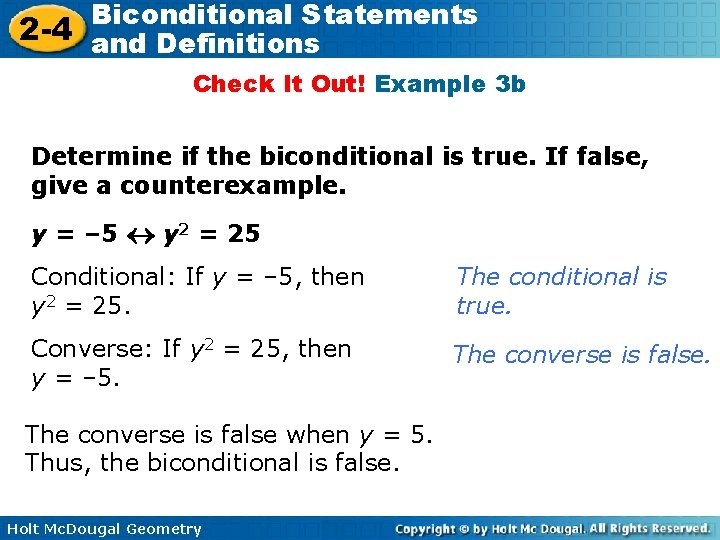Biconditional Statements 2 -4 and Definitions Check It Out! Example 3 b Determine if the biconditional is true. If false, give a counterexample. y = – 5 y 2 = 25 Conditional: If y = – 5, then y 2 = 25. The conditional is true. Converse: If y 2 = 25, then y = – 5. The converse is false when y = 5. Thus, the biconditional is false. Holt Mc. Dougal GeometryBiconditional Statements 2 -4 and Definitions In geometry, biconditional statements are used to write definitions. A definition is a statement that describes a mathematical object and can be written as a true biconditional. Holt Mc. Dougal Geometry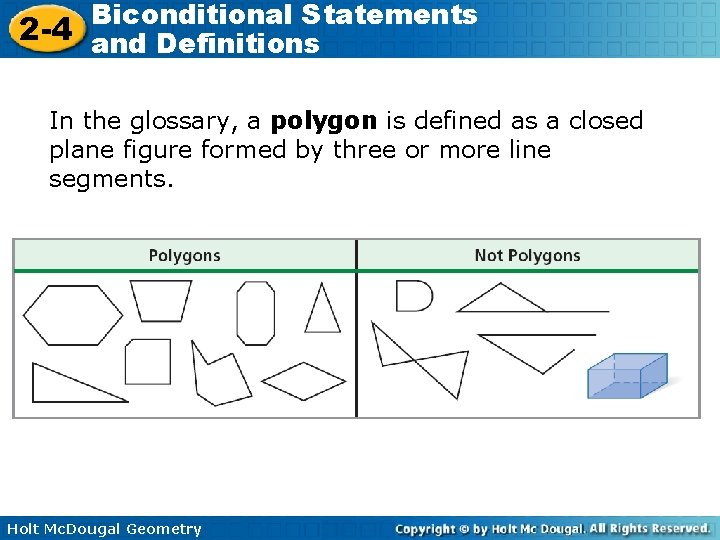Biconditional Statements 2 -4 and Definitions In the glossary, a polygon is defined as a closed plane figure formed by three or more line segments. Holt Mc. Dougal Geometry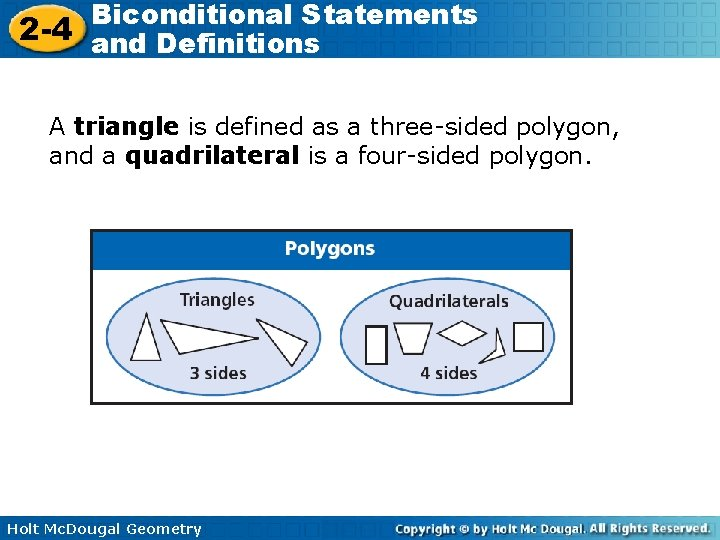Biconditional Statements 2 -4 and Definitions A triangle is defined as a three-sided polygon, and a quadrilateral is a four-sided polygon. Holt Mc. Dougal Geometry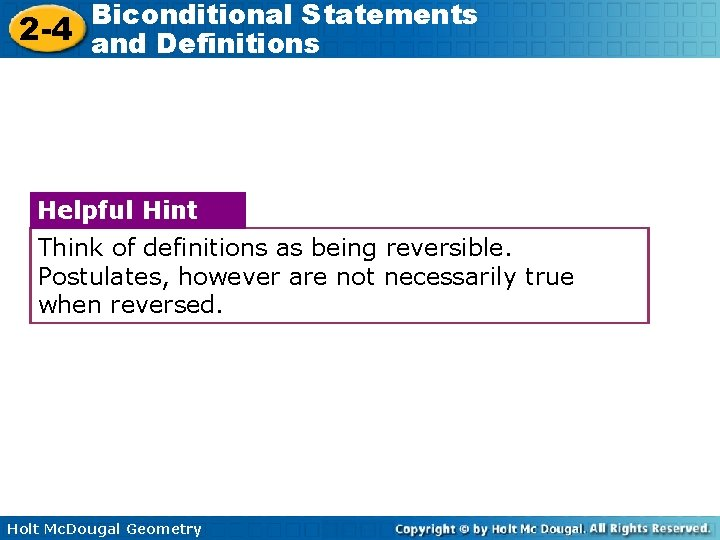Biconditional Statements 2 -4 and Definitions Helpful Hint Think of definitions as being reversible. Postulates, however are not necessarily true when reversed. Holt Mc. Dougal GeometryBiconditional Statements 2 -4 and Definitions Example 4: Writing Definitions as Biconditional Statements Write each definition as a biconditional. 1. A. A pentagon is a five-sided polygon. A figure is a pentagon if and only if it is a 5 -sided polygon. B. A right angle measures 90°. An angle is a right angle if and only if it measures 90°. Holt Mc. Dougal Geometry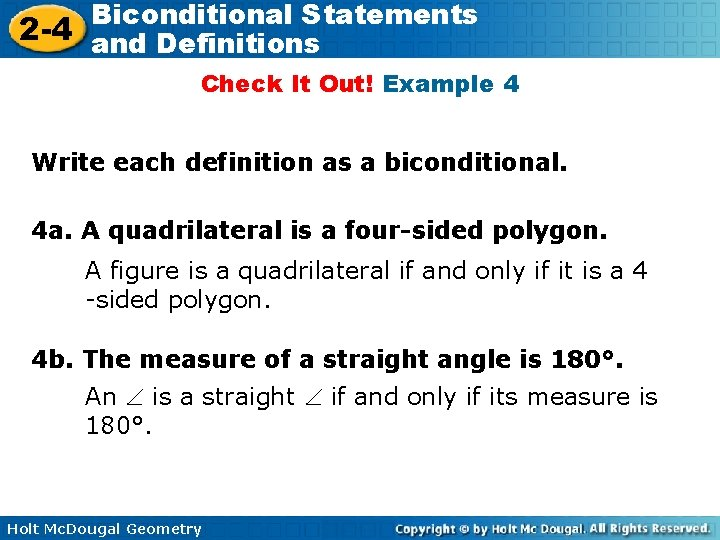Biconditional Statements 2 -4 and Definitions Check It Out! Example 4 Write each definition as a biconditional. 4 a. A quadrilateral is a four-sided polygon. A figure is a quadrilateral if and only if it is a 4 -sided polygon. 4 b. The measure of a straight angle is 180°. An is a straight if and only if its measure is 180°. Holt Mc. Dougal GeometryBiconditional Statements 2 -4 and Definitions Lesson Quiz 1. For the conditional “If an angle is right, then its measure is 90°, ” write the converse and a biconditional statement. Converse: If an measures 90°, then the is right. Biconditional: An is right iff its measure is 90°. 2. Determine if the biconditional “Two angles are complementary if and only if they are both acute” is true. If false, give a counterexample. False; possible answer: 30° and 40° 3. Write the definition “An acute triangle is a triangle with three acute angles” as a biconditional. A triangle is acute iff it has 3 acute s. Holt Mc. Dougal Geometry